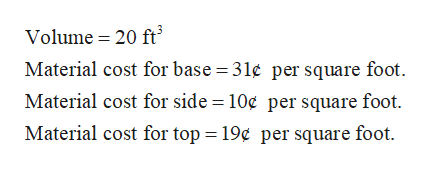# A rectangular box is to have a square base and a volume of 20 ft3. If the material for the base costs 31¢/square foot, the material for the sides costs 10¢/square foot, and the material for the top costs 19¢/square foot, determine the dimensions of the box that can be constructed at minimum cost.

Question

A rectangular box is to have a square base and a volume of 20 ft3. If the material for the base costs 31¢/square foot, the material for the sides costs 10¢/square foot, and the material for the top costs 19¢/square foot, determine the dimensions of the box that can be constructed at minimum cost.

check_circle

Step 1

Given:help_outlineImage TranscriptioncloseVolume 20 ft Material cost for base = 31¢ per square foot Material cost for side = 10¢ per square foot. Material cost for top = 19¢ per square foot fullscreen
Step 2

Let the length, width and height of the rectangular box be x, x and y respectively. Then,

Step 3

The total cost is...

### Want to see the full answer?

See Solution

#### Want to see this answer and more?

Solutions are written by subject experts who are available 24/7. Questions are typically answered within 1 hour.*

See Solution
*Response times may vary by subject and question.
Tagged in

### Calculus